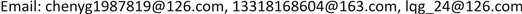1广东电网电力调度控制中心，广东 广州

2广东宝丽华电力有限公司，广东 梅州

3哈尔滨工业大学，电气工程及自动化学院，电气工程系，黑龙江 哈尔滨1. 引言

2. 需求响应模型2.1. 基于价格弹性的电价响应模型

ε = Δ q / q Δ p / p (1)

ρ i i = Δ Q e i / Q e i Δ p e i / p e i (2)

ρ i j = Δ Q e i / Q e i Δ p e j / p e j (3)

Δ P L , r , e t = P L , r , e t 0 [ E ( t , t ) ⋅ p e t − p e t 0 p e t 0 + ∑ t , g ∈ T t ≠ g E ( t , g ) ⋅ p e g − p e g 0 p e g 0 ] (4)

∑ t ∈ T r ∈ R Δ P L , r , e t = 0 (5)

− Δ P L , r , e max ≤ Δ P L , r , e t ≤ Δ P L , r , e max (6)

p e t ≥ p ¯ e (7)

2.2. 基于需求响应的热价响应模型

μ i i = Δ Q h i / Q h i Δ p h i / p h i (8)

μ i j = Δ Q h i / Q h i Δ p h j / p h j (9)

(10)

∑ t ∈ T v ∈ V Δ P L , v , h t = 0 (11)

− Δ P L , v , h max ≤ Δ P L , v , h t ≤ Δ P L , v , h max (12)

3. 计及需求响应的热电联合系统优化调度模型3.1. 需求响应参与热电联合系统风电消纳的调度框架

3.2. 计及需求响应的热电联合系统优化调度模型

3.2.1. 目标函数

min F = ∑ t = 1 T ( ∑ i = 1 N F CHP , i t + ∑ j = 1 M F CON , j t ) Δ T (13)

F CHP , i t = α i ( P CHP , i , e t ) 2 + β i ( P CHP , i , e t ) + γ i + θ i ( P CHP , i , h t ) 2 + δ i ( P CHP , i , h t ) + ξ i P CHP , i , e t P CHP , i , h t (14)

F CON , j t = a j ( P CON , j , e t ) 2 + b j ( P CON , j , e t ) + c j (15)

3.2.2. 约束条件

1) 功率平衡约束

∑ i = 1 N P CON , i , e t + ∑ j = 1 M P CHP , j , e t + ∑ k = 1 S P W P , k , e t = P L , e t (16)

2) 火电、热电和风电机组电出力约束

{ P CHP , i , e min ≤ P CHP , i , e t ≤ P CHP , i , e max P CON , j , e min ≤ P CON , j , e t ≤ P CON,j,e max P W P , k , e min ≤ P W P , k , e t ≤ P W P , k , e max (17)

3) 热电机组热出力约束

P CHP , i , h min ≤ P CHP , i , h t ≤ P CHP , i , h max (18)

4) 热电联产机组热电耦合约束

C m , i P C HP , i , h t + K i ≤ P CHP , i , e t (19)

5) 爬坡速率约束

{ − P CHP , i , e down Δ T ≤ P CHP , i , e t − P CHP , i , e t − 1 ≤ P CHP , i , e up Δ T − P CON , j , e down Δ T ≤ P CON , j , e t − P CON , j , e t − 1 ≤ P CON , j , e up Δ T − P CHP , i , h down Δ T ≤ P CHP , i , h t − P CHP , i , h t − 1 ≤ P CHP , i , h up Δ T (20)

6) 热网储热约束

P S , h t = P S , h t − 1 + ∑ j = 1 M P CHP , j , h t − P L , h t (21)

P S , h min ≤ P S , h t ≤ P S , h max (22)

7) 需求响应约束

4. 算例分析

4.1. 仿真分析

Electric and heat power output of each uni

CON1200[100, 250]0
CON2200[100, 250]0
CHP1300[150, 400][0, 500]
CHP2300[150, 400][0, 500]

Economic operation parameters of CHP unit
CHPαβγθδξC mK
17.60 × 10−50.270.754.30 × 10−50.201.14 × 10−40.7522.50
27.80 × 10−50.200.755.20 × 10−50.201.20 × 10−40.7522.50

Coal consumption parameters of CON unit
CONabc
17.20 × 10−50.229214.618
27.10 × 10−50.270511.537

4.2. 计及需求响应的热电联合系统优化调度策略优越性分析

The quantity of coal consumption and wind power curtailment under 4 strategie

A10,042.02975.843.04%
B9948.92655.338.41%
C10,008.02863.241.42%
D9918.52555.836.97%

5. 结论

1) 将电价和热价作为优化控制变量建立的电、热负荷需求响应模型能够改变用户的用电、用热行为，使得用户将自身的部分负荷需求调整到电价或者热价较低的时段，从而减小电、热负荷曲线的峰谷差。在需求响应的作用下，电价较低时段的电负荷得到增长，热价较高时段的热负荷需求降低，这就从增加电负荷和降低热电机组出力两个角度增大了风电上网空间，能够有效提高系统的风电消纳能力。

2) 与其他3种调度策略比较，考虑电、热需求响应的热电联合系统调度策略降低了系统煤耗量，使得系统经济性更佳，这对热电联合调度具有较强的现实意义。